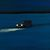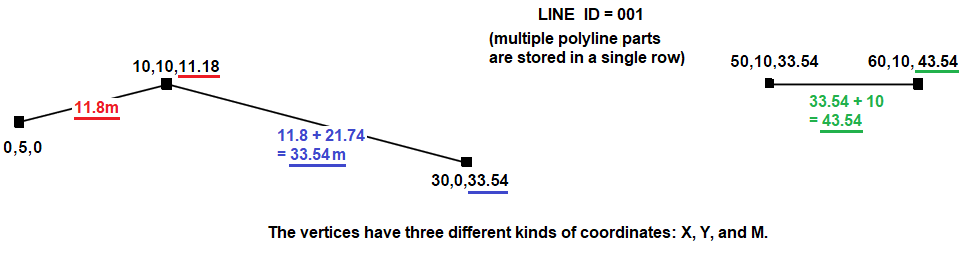# PL/SQL Code Review: Set polyline M-values to cumulative length of line

242
0
03-30-2022 07:17 AMby
Frequent ContributorI have a multi-part polyline in an SDE.ST_GEOMETRY feature class (Oracle 18c EGDB).

I've written a PL/SQL function that replaces the geometry's M-values with the cumulative length of the line.
(My plan is to eventually create a database trigger that uses the function to update polyline geometries — when features get edited.)

```Input:
MULTILINESTRING M (( 0.0 5.0 -100000.0, 10.0 10.0 -100000.0, 30.0 0.0 -123),( 50.0 10.0 456, 60.0 10.0 789))
--select sde.st_astext(shape) from polylines

Output:
MULTILINESTRING M ((0 5 0, 10 10 11.18, 30 0 33.54),(50 10 33.54, 60 10 43.54))
--select m_as_length(shape) from polylines```

Question:

The m_as_length() function works as expected.
But I'm a PL/SQL novice, so I thought I'd ask: Can the script be improved?

```with
function pythagoras(x1 in number, y1 in number, x2 in number, y2 in number) return number is
begin
return round(sqrt( (x2 - x1) * (x2 - x1) + (y2 - y1) * (y2 - y1)), 2);  -- Power is a slow function
end;

function m_as_length(shape in sde.st_geometry) return varchar2
is
result              varchar2(32767);
vertex_set          varchar2(32767);
oldX                number;
oldY                number;
newX                number;
newY                number;
line_len            number := 0;
begin
for vPartIndex in 1..sde.st_geometry_operators.st_numgeometries_f(shape)
loop
vertex_set      := null;
for vPointIndex in 1..sde.st_geometry_operators.st_numpoints_f(sde.st_geometry_operators.ST_GeometryN_f(shape,vPartIndex))
loop
newX        := sde.st_geometry_operators.st_x_f(sde.st_geometry_operators.st_pointn_f(sde.st_geometry_operators.st_geometryn_f(shape,vPartIndex),vPointIndex));
newY        := sde.st_geometry_operators.st_y_f(sde.st_geometry_operators.st_pointn_f(sde.st_geometry_operators.st_geometryn_f(shape,vPartIndex),vPointIndex));
if vPointIndex <> 1 then
line_len := line_len + pythagoras(oldX, oldY, newX, newY);
end if;
oldX        := newX;
oldY        := newY;
vertex_set  := vertex_set || newX || ' ' || newY || ' ' || line_len || ', ';
end loop;
result          := result || '(' || rtrim((vertex_set),', ') || '),';
end loop;
return 'MULTILINESTRING M (' || rtrim((result),',') || ')';
end;
select
m_as_length(shape)
from
polyline```

Related:

1. Use SDE.ST_GEOMETRY functions in a custom function

• Explains why the function calls are so verbose:  sde.st_geometry_operators.st_numgeometries_f.

2. ST_GEOMETRY functions:

3. I'm aware that this sort of thing can be achieved with Arcade in ArcGIS Pro 2.6+. Even still, I want to learn how to do this in PL/SQL, for professional development reasons, and to have a plan B, for scenarios where Arcade won't work (due to compatibility issues, etc.).

4. My FC is registered as versioned (with option to move edits to base). We don't use named versions, we only use the default version (so that we can undo/redo edits while editing). So in this case, using database triggers on a versioned FC shouldn't be an issue -- especially since we're exclusively working in the base FC -- and are using the "with base" option.

0 Replies